# Insertion Sort

Updated:

## 1. Pseudocode

-- Interactive version --
algorithm InsertionSort(array, n):
For j := 2 to n do
key := array[j]
i := j - 1
while i > 0 and array[i] > key:
array[i] = array[i+1]
i -= 1
arrary[i+1] = key

-- Recursive version --
algorithm InsertionRec(A): --> A == array
InsertionRecAux(A, A.length) --> arbitrarily take the last element as the key

algorithm InsertionRecAux(A, keyIndex):
if keyIndex <= 1 then
return
InsertionRecAux(A, keyIndex-1)
Insert(A, keyIndex)

algorithm Insert(A, keyIndex):
key := A[keyIndex]
i := keyIndex - 1
While i > 0 and A[i] > key do
A[i+1] = A[i]
i -= 1
A[i+1] := key


Remark on the recursive version:

• In the Insert() phase, we assume that the input-array A is already sorted. This is because we recursively call InsertionRecAux() and the call-stack only bounces back (return) when it hits the base case, which is, in this case, when keyIndex <= 1.
• When we start inserting, therefore, the index of the key starts from 1 (one element per se is sorted)
• In other words, we do recursion first and only start inserting when recursion stack bounces back.

## 2. Time cmplexity

### (1) Upper bound

$T(n) = for + for \cdot while \approx c_0\cdot n + c_1\cdot\textstyle\sum_2^n(j-1)$ $T(n) \in O(n^2)$

### (2) Θ and O

#### Worst-case time complexity of insertion sort is in

$O(n^2)\space or\space \Theta(n^2)$ but the time complexity of insertion sort is in $O(n^2)$ but NOT in $Θ(n^2)$ Recall the definiation of Theta and O()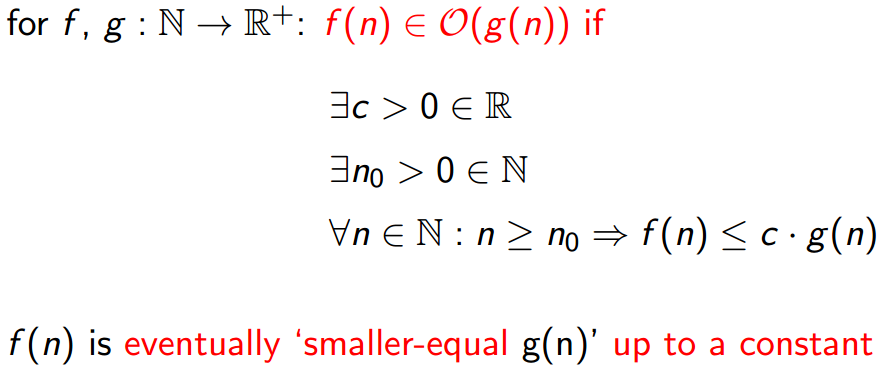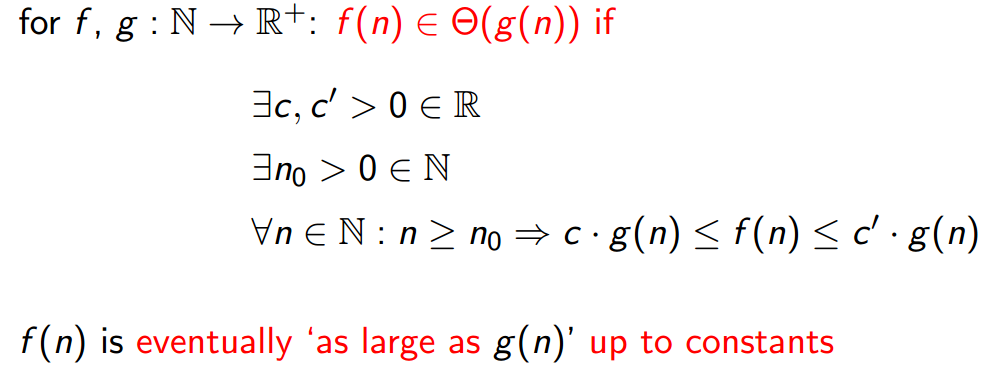the best-case of insertion sort $Θ(n^2)$

## \$ Worst-case in-depth analysis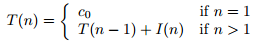where I(n) gives the running time for inserting an element (‘the nth element’) in a sorted array of length (n − 1). Inserting an element at the right position in a sorted array of length (n − 1) takes in the worst-case order n steps because then we have to traverse the complete array. So I(n) = c1 · n + c2 for some constants c1 and c2. Hence we find for the recurrence equation for T the following: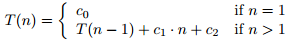Using the substitution method we find: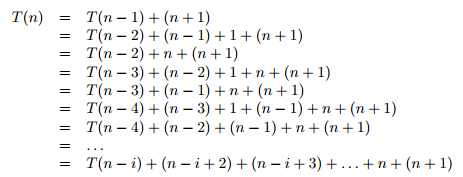We hit the base case when n − i = 1, that is, i = n − 1. We then substitute and find: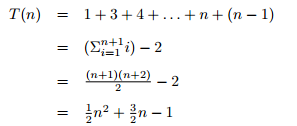Hence, T(n) is in Theta(n^2^)

Tips: Compared with marge sort, for insertion sort, we have a recursive call on the array with only one element remains.

## 3. Space complexity

Insertion sort is an in-place sorting algorithm.

## 4. Code

# python:
def InsertionSort(array, n):
for j in range(n):
key = array[j]
i = j - 1
while i >= 0 and array[i] > key:
array[i+1] = array[i]
i -= 1
array[i+1]  = key

if __name__ == "__main__":
array = [4234,234234,23,234,-134,4123,41,578,-342,55,0.2323,0.325]
print("\nBefore sorting:", array)
InsertionSort(array, len(array))
print("\nAfter sorting:", array)

// Scala:
object InsersionSortAlgo {

def InsersionSort(array: Array[Double], n: Int): Array[Double] = {
for (j <- 1 until n) {
var key: Double = array(j)
var i: Int = j - 1
while (i >= 0 && array(i) > key) {
array(i+1) = array(i)
i -= 1
}
array(i+1) = key
}
array
}

def main(args:Array[String]): Unit = {
var array = Array(4234,234234,23,234,-134,4123,41,578,-342,55,0.2323,0.325)
println("\nBefore sorting:")
println(array.deep.mkString(" "))
println("\nAfter sorting:")
println(InsersionSort(array, array.length).deep.mkString(" "))
}
}


Tags: